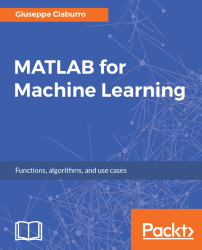•#### MATLAB for Machine Learning#### Overview of this book

MATLAB is the language of choice for many researchers and mathematics experts for machine learning. This book will help you build a foundation in machine learning using MATLAB for beginners. You’ll start by getting your system ready with t he MATLAB environment for machine learning and you’ll see how to easily interact with the Matlab workspace. We’ll then move on to data cleansing, mining and analyzing various data types in machine learning and you’ll see how to display data values on a plot. Next, you’ll get to know about the different types of regression techniques and how to apply them to your data using the MATLAB functions. You’ll understand the basic concepts of neural networks and perform data fitting, pattern recognition, and clustering analysis. Finally, you’ll explore feature selection and extraction techniques for dimensionality reduction for performance improvement. At the end of the book, you will learn to put it all together into real-world cases covering major machine learning algorithms and be comfortable in performing machine learning with MATLAB.
Title PageCreditsForewordwww.PacktPub.comCustomer FeedbackPrefaceFree Chapter
Getting Started with MATLAB Machine LearningImporting and Organizing Data in MATLABFrom Data to Knowledge DiscoveryFinding Relationships between Variables - Regression TechniquesPattern Recognition through Classification AlgorithmsIdentifying Groups of Data Using Clustering MethodsSimulation of Human Thinking - Artificial Neural NetworksImproving the Performance of the Machine Learning Model - Dimensionality ReductionMachine Learning in Practice## Searching linear relationships

In the previous chapter, we learned that the coefficient of correlation between two quantitative variables, X and Y, provides information on the existence of a linear relation between the two variables. This index, however, does not allow determining whether it is X that affects Y, or it is Y that affects X, or whether both X and Y are consequences of a phenomenon that affects both of them. Only more knowledge of the problem under study can allow some hypothesis of the dependence of a variable on another.

### Note

If a correlation between two variables is not found, it does not necessarily imply that they are independent, because they might have a nonlinear relationship.

Calculating correlation and covariance is a useful way to investigate whether a linear relationship exists between variables, without having to assume or fit a specific model to our data. It can happen that two variables have a small or no linear correlation, meaning a strong nonlinear relationship...